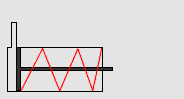Home » Pneumatic Cylinder Air Flow Calculation
Instrumentation Design

Pneumatic Cylinder Air Flow Calculation

This is critical to ensure that the pneumatic cylinder is supplied with enough air flow to operate as it is intended in your system design. This calculation does depend on the type of cylinder you are using, particularly whether it is a single-acting cylinder or a double-acting cylinder.

Pneumatic Cylinder Information

First, we must gather some basic information about your pneumatic cylinder so we can calculate the air consumption:

• Piston Area (Bore x 3.1415)
• Rod Area (for double-acting cylinders)
• Stroke
• Cycles per minute

Air Flow Required for Single-Acting CylinderCalculating the air consumption for a single-acting cylinder is a rather easy calculation, but it is important to understand that the required air flow is dependent not only on the bore area and stroke, but also the cycle rate of the pneumatic cylinder.

The formula for the air consumption of a single-acting cylinder is as follows:

• A = Piston Area (Square Inches)
• S = Stroke (Inches)
• C = Cycles per Minute
• CFM (Cubic Feet per Minute) = (A x S x C) / 1728

Keep in mind that dwell times and cylinder sequence can affect the results when using this formula.

Air Flow Required for Double-Acting CylinderIn a double-acting cylinder, the retracting volume and extending volume differ by the displacement of the rod.

The formula for the air consumption of a double-acting pneumatic cylinder is as follows:

• A = Piston Area (Square Inches)
• R= Rod Area (Square Inches)
• S = Stroke (Inches)
• C = Cycles per Minute
• CFM (Cubic Feet per Minute) = [(2*A – R) x S x C] / 1728

Keep in mind that dwell times and cylinder sequence can affect the results when using this formula.

In certain applications, the double-acting cylinder may be required to extend and retract at a different rate. Obviously, this changes our calculation of the air consumption.

In this situation, the air consumption of each stroke must be calculated separately as follows:

• EC = Extend cycles per minute (ignoring dwell time)
• RC = Retract cycles per minute (ignoring dwell time)
• CFM =  (A x S x EC) / 1728 + [(A – R) x S x RC] / 1728

Converting CFM to SCFM

Converting the flow rate from CFM (compressed air) to SCFM (free air) can be calculated as follows:

• P = Pressure (PSIG)
• SCFM = CFM x (P + 14.7) / 14.7

Knowing how to use these calculations help with the sizing of the system components as well as calculation of the total air consumption of the system.

Credits : Ryan Christian

Source : cross-automation.com

How-to Create Instrument Loop Diagram (ILD) ?

This website uses cookies to improve your experience. We'll assume you're ok with this, but you can opt-out if you wish. Accept Read More

WordPress Image Lightbox

Send this to a friend